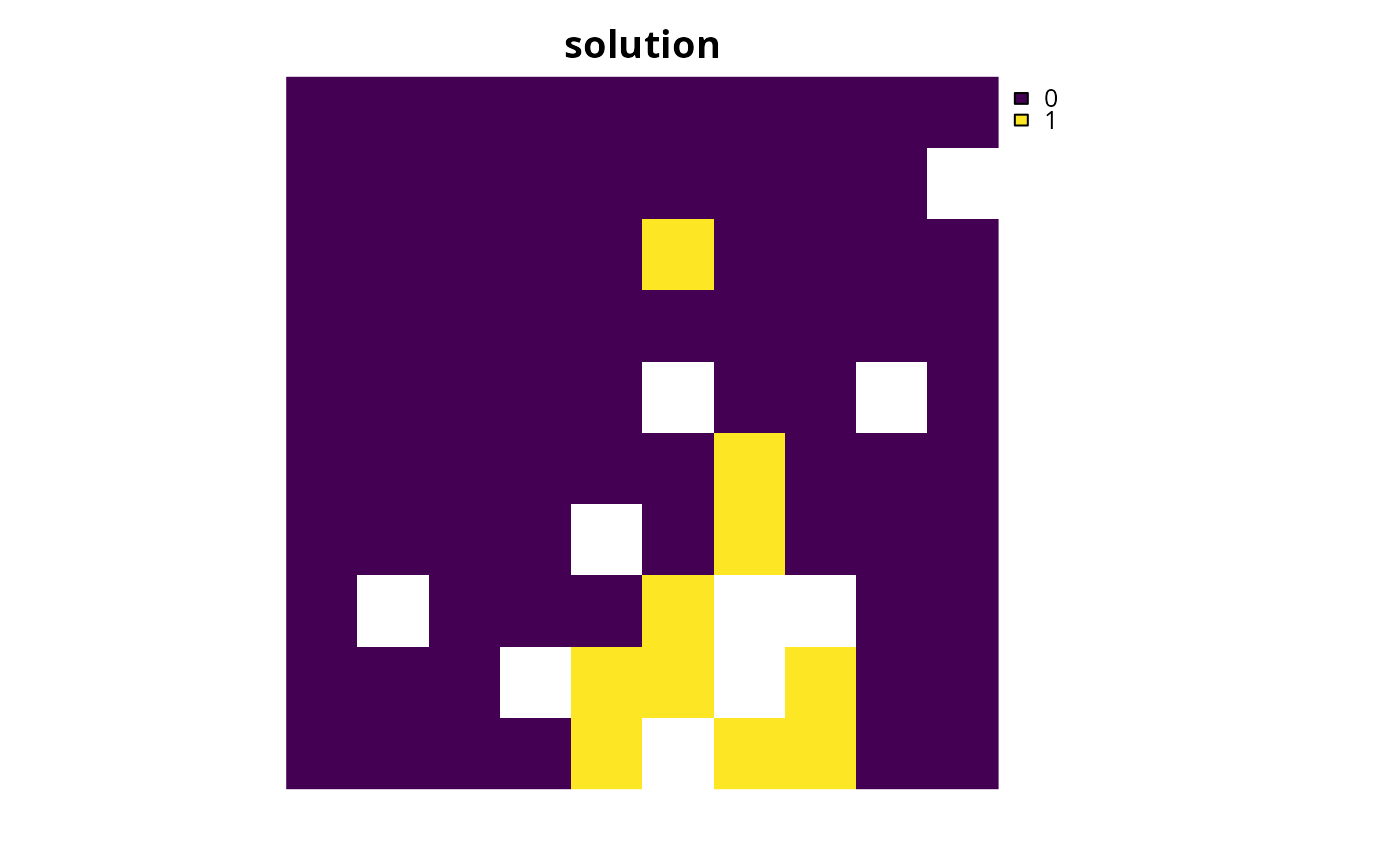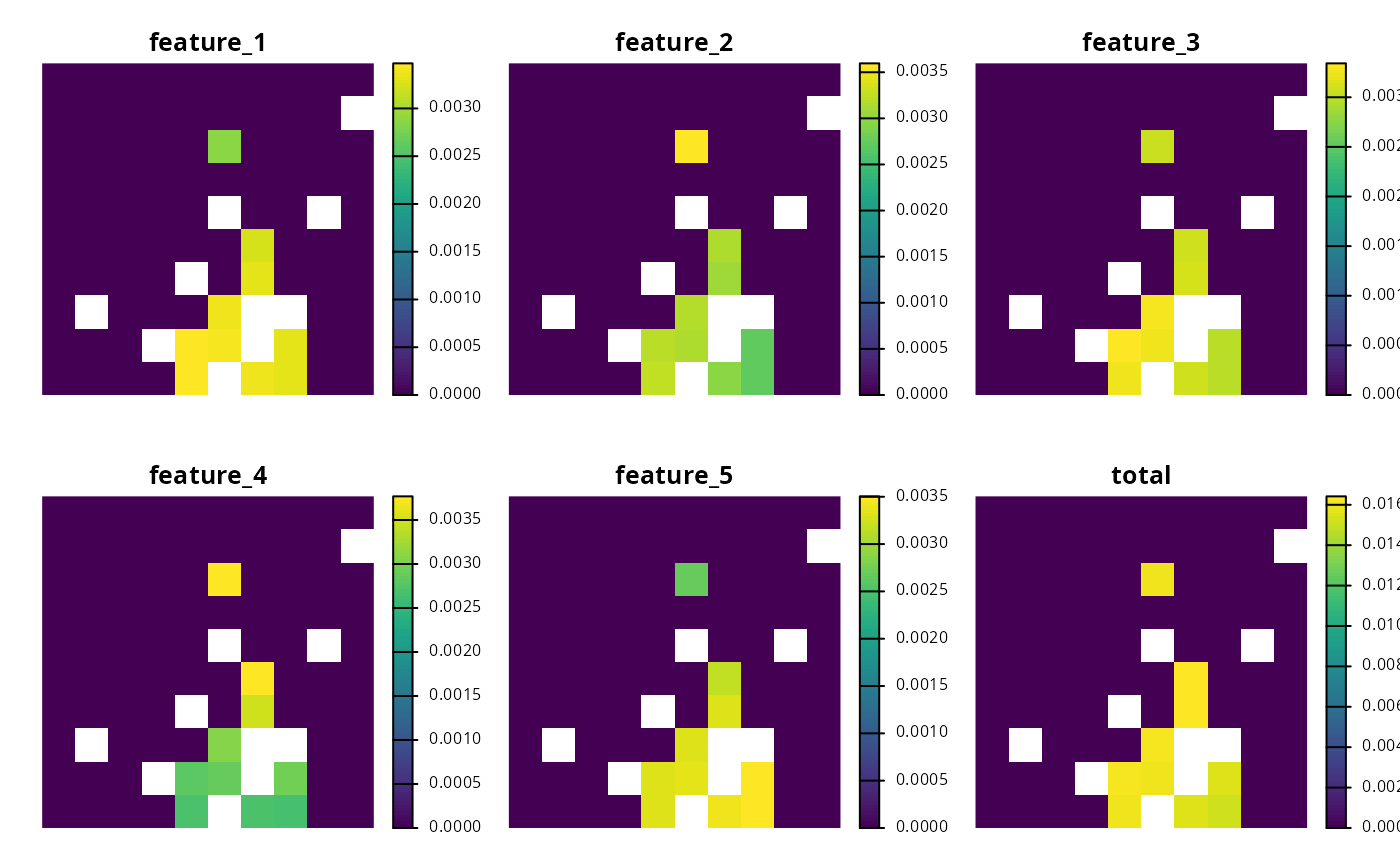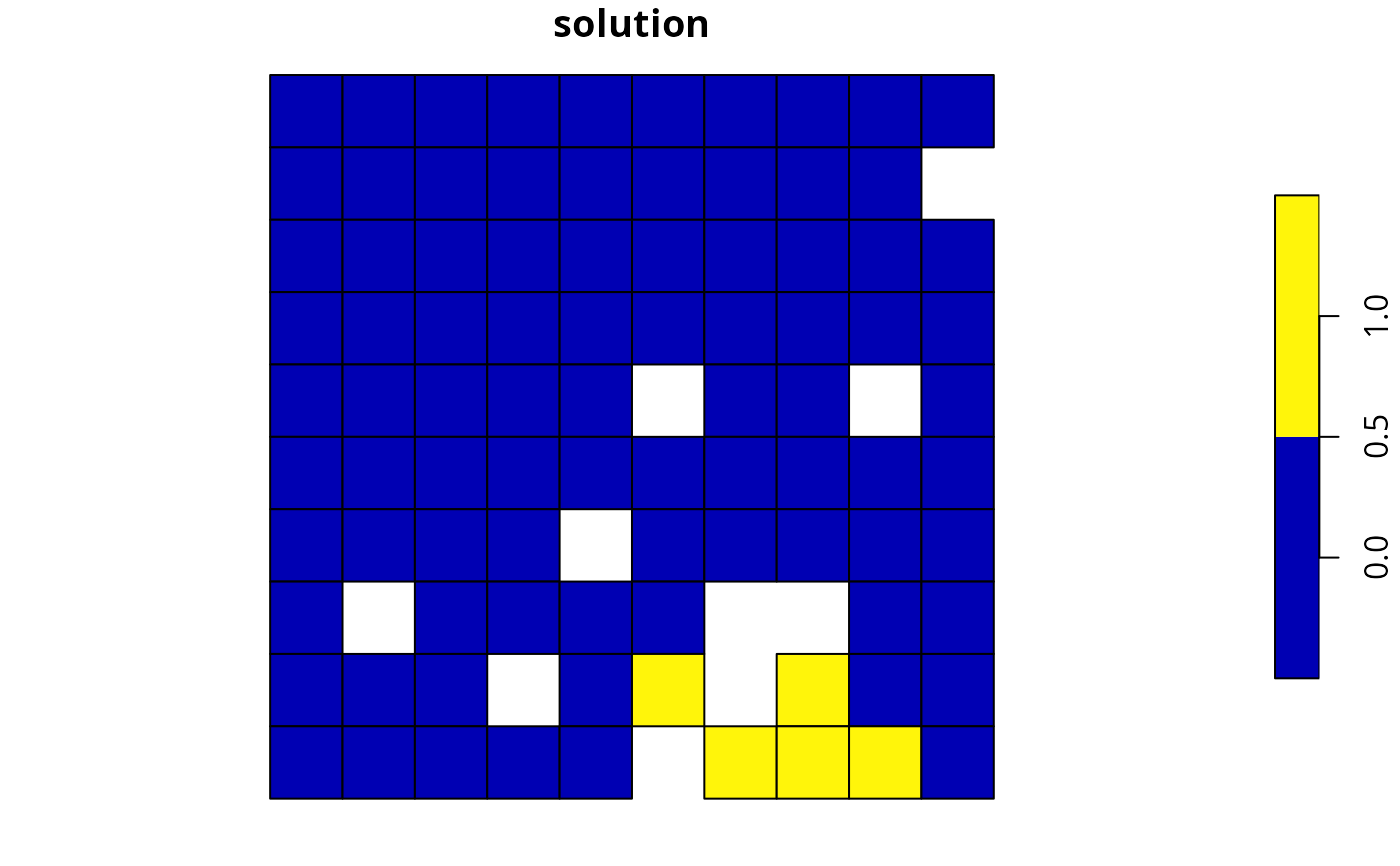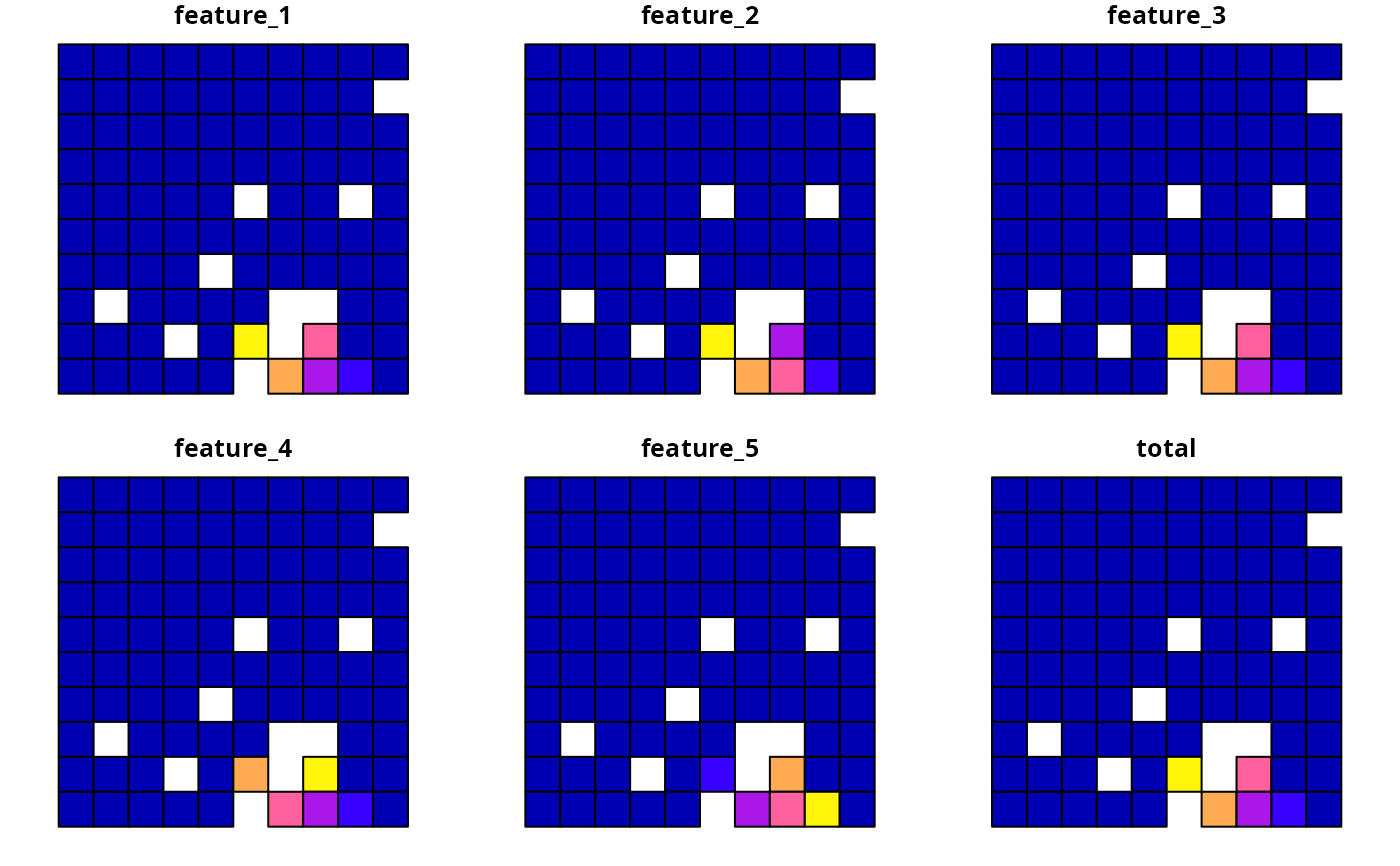Calculate importance scores for planning units selected in a solution following Ferrier et al. (2000).

eval_ferrier_importance(x, solution)

# S4 method for ConservationProblem,numeric
eval_ferrier_importance(x, solution)

# S4 method for ConservationProblem,matrix
eval_ferrier_importance(x, solution)

# S4 method for ConservationProblem,data.frame
eval_ferrier_importance(x, solution)

# S4 method for ConservationProblem,Spatial
eval_ferrier_importance(x, solution)

# S4 method for ConservationProblem,sf
eval_ferrier_importance(x, solution)

# S4 method for ConservationProblem,Raster
eval_ferrier_importance(x, solution)

## Arguments

x

problem() (i.e., ConservationProblem) object.

solution

numeric, matrix, data.frame, Raster, Spatial, or sf::sf() object. The argument should be in the same format as the planning unit cost data in the argument to x. See the Solution format section for more information.

## Value

A matrix, tibble::tibble(), RasterLayer, or Spatial object containing the scores for each planning unit selected in the solution. Specifically, the returned object is in the same format (except if the planning units are a numeric vector) as the planning unit data in the argument to x.

## Details

Importance scores are reported separately for each feature within each planning unit. Additionally, a total importance score is also calculated as the sum of the scores for each feature. Note that this function only works for problems with a minimum set objective and a single zone. It will throw an error for problems that do not meet this criteria.

## Solution format

Broadly speaking, the argument to solution must be in the same format as the planning unit data in the argument to x. Further details on the correct format are listed separately for each of the different planning unit data formats:

x has numeric planning units

The argument to solution must be a numeric vector with each element corresponding to a different planning unit. It should have the same number of planning units as those in the argument to x. Additionally, any planning units missing cost (NA) values should also have missing (NA) values in the argument to solution.

x has matrix planning units

The argument to solution must be a matrix vector with each row corresponding to a different planning unit, and each column correspond to a different management zone. It should have the same number of planning units and zones as those in the argument to x. Additionally, any planning units missing cost (NA) values for a particular zone should also have a missing (NA) values in the argument to solution.

x has Raster planning units

The argument to solution be a Raster object where different grid cells (pixels) correspond to different planning units and layers correspond to a different management zones. It should have the same dimensionality (rows, columns, layers), resolution, extent, and coordinate reference system as the planning units in the argument to x. Additionally, any planning units missing cost (NA) values for a particular zone should also have missing (NA) values in the argument to solution.

x has data.frame planning units

The argument to solution must be a data.frame with each column corresponding to a different zone, each row corresponding to a different planning unit, and cell values corresponding to the solution value. This means that if a data.frame object containing the solution also contains additional columns, then these columns will need to be subsetted prior to using this function (see below for example with sf::sf() data). Additionally, any planning units missing cost (NA) values for a particular zone should also have missing (NA) values in the argument to solution.

x has Spatial planning units

The argument to solution must be a Spatial object with each column corresponding to a different zone, each row corresponding to a different planning unit, and cell values corresponding to the solution value. This means that if the Spatial object containing the solution also contains additional columns, then these columns will need to be subsetted prior to using this function (see below for example with sf::sf() data). Additionally, the argument to solution must also have the same coordinate reference system as the planning unit data. Furthermore, any planning units missing cost (NA) values for a particular zone should also have missing (NA) values in the argument to solution.

x has sf::sf() planning units

The argument to solution must be a sf::sf() object with each column corresponding to a different zone, each row corresponding to a different planning unit, and cell values corresponding to the solution value. This means that if the sf::sf() object containing the solution also contains additional columns, then these columns will need to be subsetted prior to using this function (see below for example). Additionally, the argument to solution must also have the same coordinate reference system as the planning unit data. Furthermore, any planning units missing cost (NA) values for a particular zone should also have missing (NA) values in the argument to solution.

See importance for an overview of all functions for evaluating the importance of planning units selected in a solution.

Other importances: eval_rare_richness_importance(), eval_replacement_importance()

## Examples

# seed seed for reproducibility
set.seed(600)

data(sim_pu_raster, sim_features)

# create minimal problem with binary decisions
p1 <- problem(sim_pu_raster, sim_features) %>%
add_default_solver(gap = 0, verbose = FALSE)
# \dontrun{
# solve problem
s1 <- solve(p1)

# print solution
print(s1)
#> class      : RasterLayer
#> dimensions : 10, 10, 100  (nrow, ncol, ncell)
#> resolution : 0.1, 0.1  (x, y)
#> extent     : 0, 1, 0, 1  (xmin, xmax, ymin, ymax)
#> crs        : NA
#> source     : memory
#> names      : layer
#> values     : 0, 1  (min, max)
#>

# plot solution
plot(s1, main = "solution", axes = FALSE, box = FALSE)# calculate importance scores using Ferrier et al. 2000 method
fs1 <- eval_ferrier_importance(p1, s1)

# print importance scores,
# each planning unit has an importance score for each feature
# (as indicated by the column names) and each planning unit also
# has an overall total importance score (in the "total" column)
print(fs1)
#> class      : RasterStack
#> dimensions : 10, 10, 100, 6  (nrow, ncol, ncell, nlayers)
#> resolution : 0.1, 0.1  (x, y)
#> extent     : 0, 1, 0, 1  (xmin, xmax, ymin, ymax)
#> crs        : NA
#> names      :     layer.1,     layer.2,     layer.3,     layer.4,     layer.5,       total
#> min values :           0,           0,           0,           0,           0,           0
#> max values : 0.003472042, 0.003596401, 0.003341572, 0.003768489, 0.003504223, 0.016417187
#>

# plot total importance scores
plot(fs1, main = "Ferrier scores", axes = FALSE, box = FALSE)# create minimal problem with polygon (sf) planning units
p2 <- problem(sim_pu_sf, sim_features, cost_column = "cost") %>%
add_default_solver(gap = 0, verbose = FALSE)

# solve problem
s2 <- solve(p2)

# print solution
print(s2)
#> Simple feature collection with 90 features and 4 fields
#> Geometry type: POLYGON
#> Dimension:     XY
#> Bounding box:  xmin: 0 ymin: 0 xmax: 1 ymax: 1
#> CRS:           NA
#> First 10 features:
#>        cost locked_in locked_out solution_1                       geometry
#> 1  215.8638     FALSE      FALSE          0 POLYGON ((0 1, 0.1 1, 0.1 0...
#> 2  212.7823     FALSE      FALSE          0 POLYGON ((0.1 1, 0.2 1, 0.2...
#> 3  207.4962     FALSE      FALSE          0 POLYGON ((0.2 1, 0.3 1, 0.3...
#> 4  208.9322     FALSE       TRUE          0 POLYGON ((0.3 1, 0.4 1, 0.4...
#> 5  214.0419     FALSE      FALSE          0 POLYGON ((0.4 1, 0.5 1, 0.5...
#> 6  213.7636     FALSE      FALSE          0 POLYGON ((0.5 1, 0.6 1, 0.6...
#> 7  210.4612     FALSE      FALSE          0 POLYGON ((0.6 1, 0.7 1, 0.7...
#> 8  211.0424     FALSE       TRUE          0 POLYGON ((0.7 1, 0.8 1, 0.8...
#> 9  210.3878     FALSE      FALSE          0 POLYGON ((0.8 1, 0.9 1, 0.9...
#> 10 204.3971     FALSE      FALSE          0 POLYGON ((0.9 1, 1 1, 1 0.9...

# plot solution
plot(s2[, "solution_1"], main = "solution")# calculate importance scores
fs2 <- eval_ferrier_importance(p2, s2[, "solution_1"])

# plot importance scores
plot(fs2, main = "Ferrier scores")# }